#Math Worksheets

## Dynamically Created Math Worksheets

Teachers, please share the site with Parents so the students can continue on with math while at home.

Math-Aids.Com provides free math worksheets for teachers, parents, students, and home schoolers. The math worksheets are randomly and dynamically generated by our math worksheet generators. This allows you to make an unlimited number of printable math worksheets to your specifications instantly.

The website contains over 94 different math topics with over 1223 unique worksheets.
These math worksheets are a great resource for Kindergarten through 12th grade.
They may be customized to fit your needs and may be printed immediately or saved for later use. These math worksheets are randomly created by our math worksheet generators, so you have an endless supply of quality math worksheets at your disposal. These high quality math worksheets are delivered in a PDF format and includes the answer keys. Our math worksheets are free to download, easy to use, and very flexible. A detailed description is provided in each math worksheets section.

The flexibility and text book quality of the math worksheets, makes Math-Aids.Com a very unique resource for people wanting to create and use math worksheets. The answer key is included with the math worksheets as it is created. Each math topic has several different types of math worksheets to cover various types of problems you may choose to work on.

We are dedicated in building the best dynamic Math Worksheets for our users.

We currently have math worksheets for the following topics, Addition, Algebra 1, Algebra 2, Decimals, Division, Estimation, Even and Odd, Exponents, Fact Family, Factors, Flash Cards, Fractions, Function Tables, Geometry, Graph, Graph Paper, Graphing, Greater Than Less Than, Hundreds Chart, In and Out Boxes, Integers, Kindergarten, Logic, Mean Mode Median & Range, Measurement, Mixed Problems, Money, Multiplication, Number Bonds, Number Lines, Number Systems, Order of Operations, Patterns, Percent, Place Value, Pre-Algebra, Probability, Properties, Pythagorean Theorem, Radicals, Ratios, Rounding, Significant Figures, Skip Counting, Subtraction, Telling Time, Venn Diagrams, Word Games and Word Problems.

We are adding new math worksheets to the site every day so visit us often. We will be glad to design any math worksheets you might need for your Lesson Planning. Just  Contact Us, we will be happy to assist you.

Teachers and Home schoolers use the math worksheets on this website to measure the children's mastery of basic math skills, give extra practice, homework practice, and save precious planning time.

Parents use the math worksheets on this website to give their children extra practice with essential math skills. Using the math worksheets over breaks and during the summer will allow children to stay sharp and get ready for the upcoming school term.

If you like our Math Worksheets and can link this website on any web page, blog, classroom site, or school resource site, we would really appreciate it!

Every page or blog that links to us is a vote that matters in the eyes of the search engines, and it is the best way to pay us a compliment.

If you find that our dynamically created math worksheets on Math-Aids.Com to be valuable to you personally, please bookmark it and share it with your friends, family, and colleagues by emailing them the site.

You can also share the site on Facebook, Twitter, Google, Pinterest, Linkedin, Wordpress, Digg, Diigo, Blogger, Stumble Upon, Tumblr, Delicious, MySpace or any social network. Simply use the buttons either on the left under the Share The Site heading or the buttons below.

## Quick Link for All Math Worksheet Sections

Click the image to be taken to that Math Worksheet Section.##### Algebra 1 WorksheetsMath Worksheets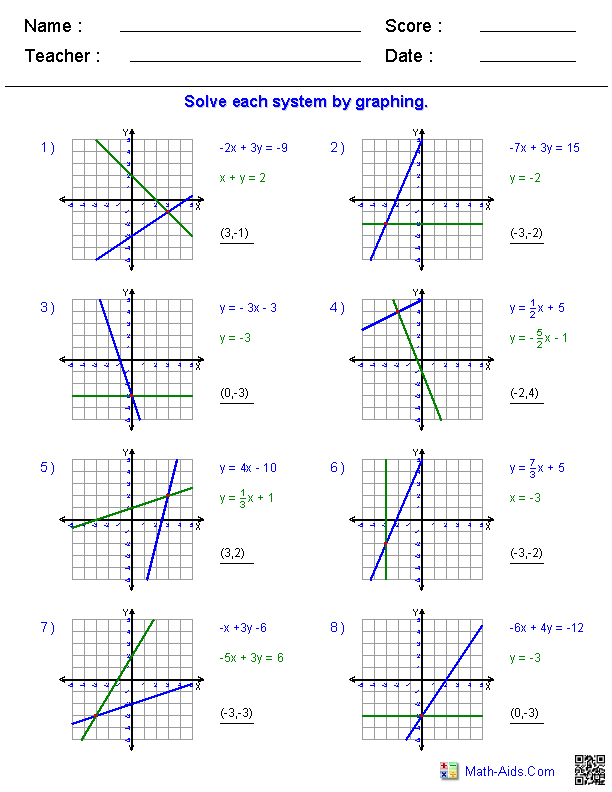##### Algebra 2 WorksheetsMath Worksheets##### Calculus WorksheetsMath Worksheets##### Decimals WorksheetsMath Worksheets##### Division WorksheetsMath Worksheets##### Estimation WorksheetsMath Worksheets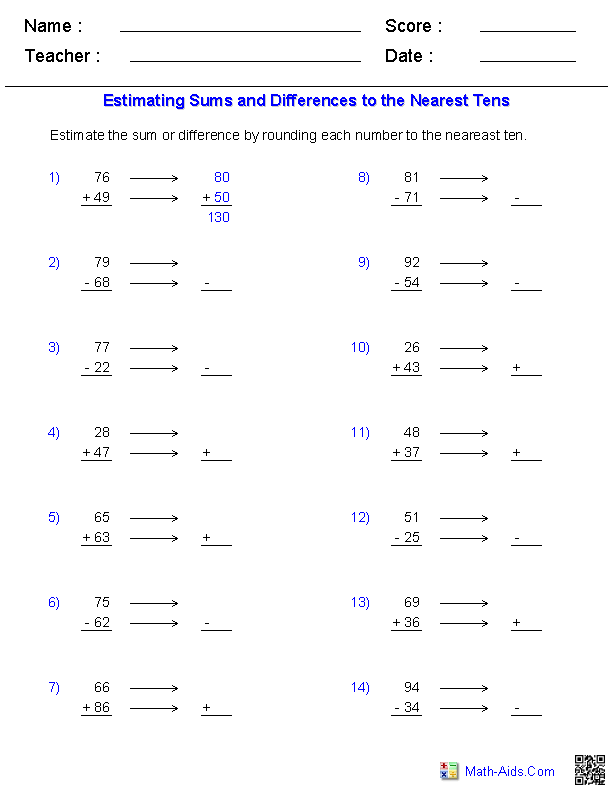##### Even and Odd WorksheetsMath Worksheets##### Exponents WorksheetsMath Worksheets##### Fact Family WorksheetsMath Worksheets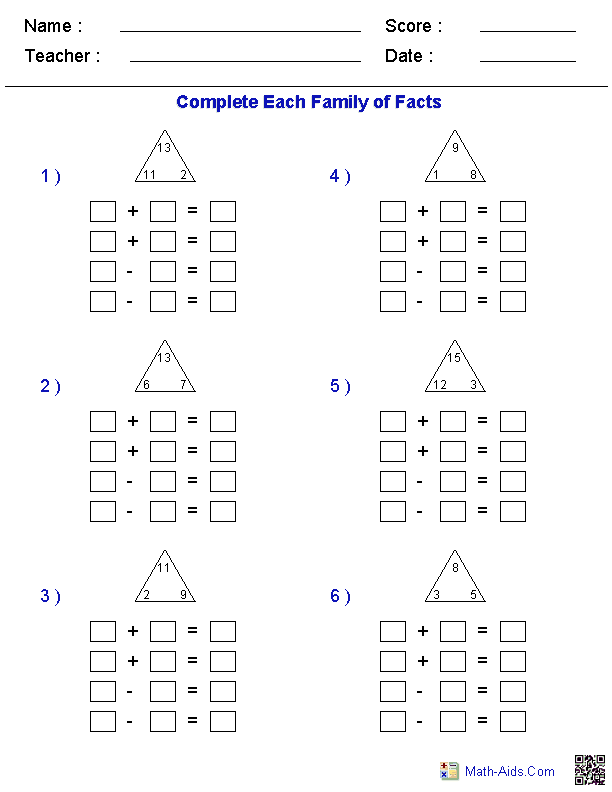##### Factors WorksheetsMath Worksheets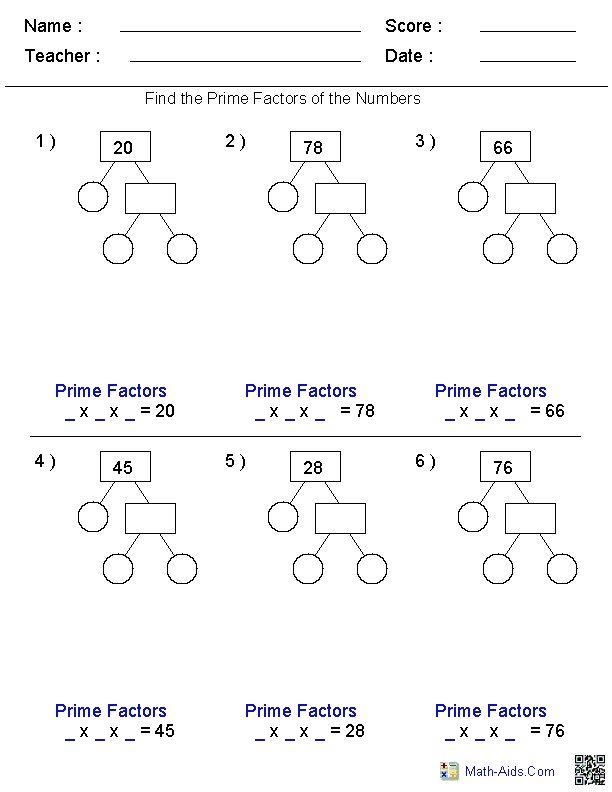##### Math Flash CardsMath Worksheets##### Fractions WorksheetsMath Worksheets##### Function Table WorksheetsMath Worksheets##### Geometry WorksheetsMath Worksheets##### GraphMath Worksheets##### Graph PaperMath Worksheets##### Graphing WorksheetsMath Worksheets##### Greater ThanLess Than Worksheets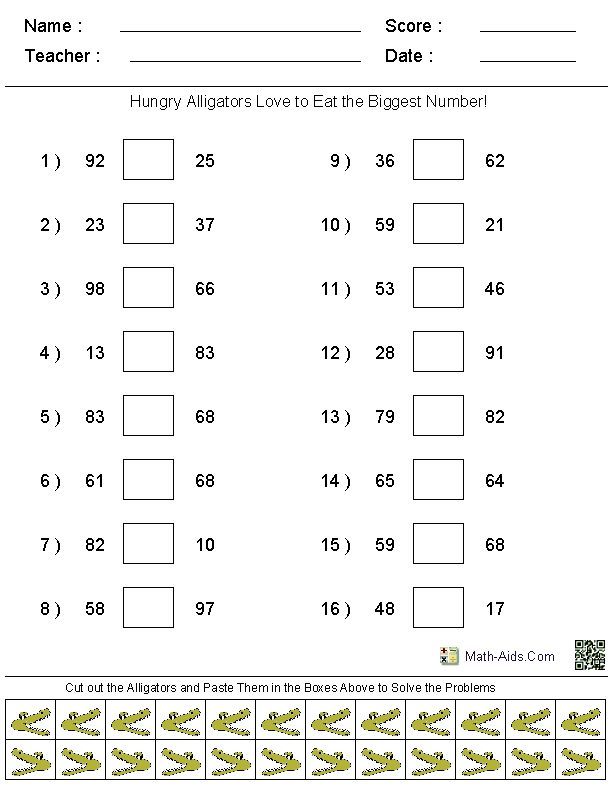##### Hundreds ChartMath Worksheets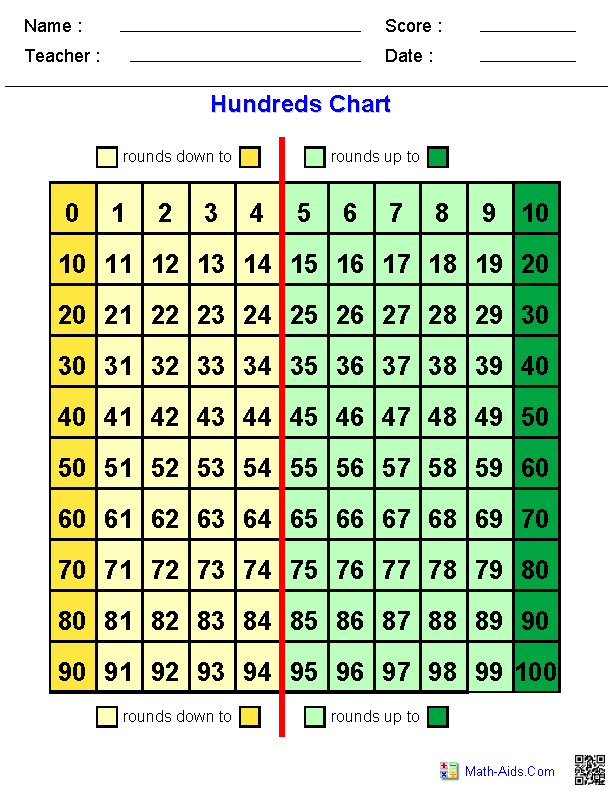##### In and Out Boxes WorksheetsMath Worksheets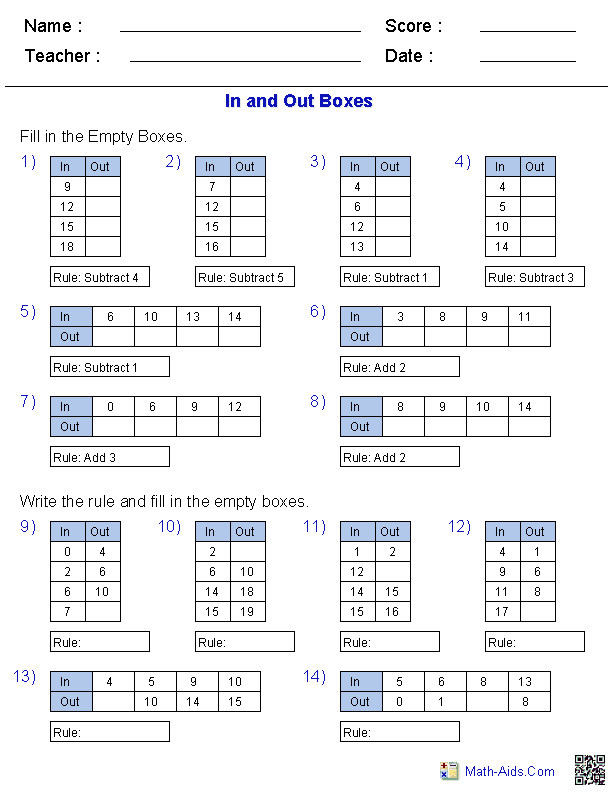##### Integers WorksheetsMath Worksheets##### Kindergarten WorksheetsMath Worksheets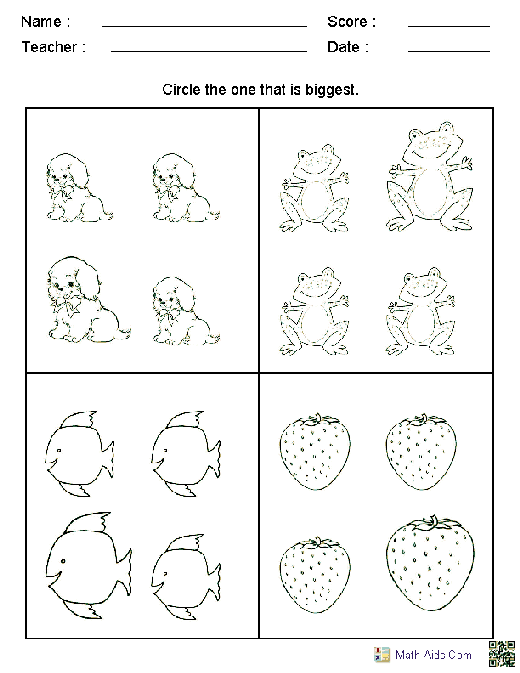##### Mean Mode Median and Range Worksheets##### Measurement WorksheetsMath Worksheets##### Mixed Problems WorksheetsMath Worksheets##### Money WorksheetsMath Worksheets##### Multiplication WorksheetsMath Worksheets##### Number Bonds WorksheetsMath Worksheets##### Number Lines WorksheetsMath Worksheets##### Number Systems WorksheetsMath Worksheets##### Order of OperationsMath Worksheets##### Patterns WorksheetsMath Worksheets##### Percent WorksheetsMath Worksheets##### Place Value WorksheetsMath Worksheets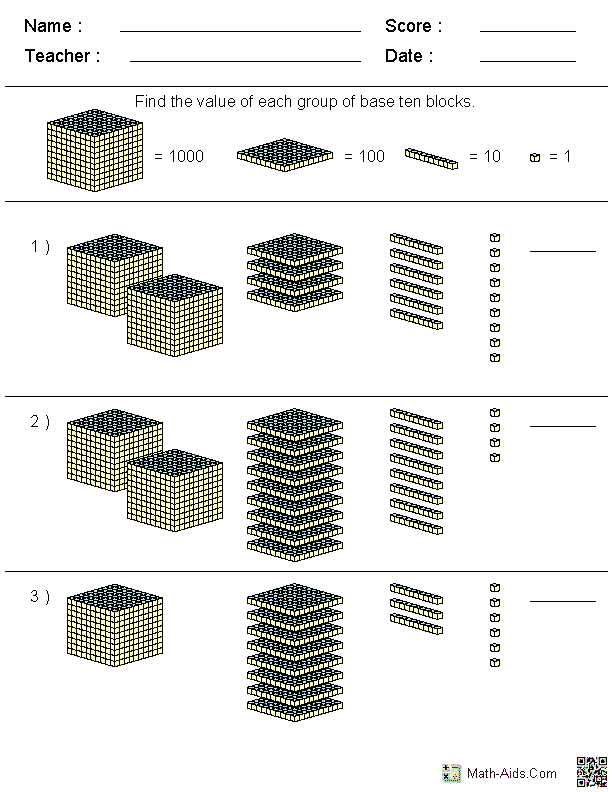##### Pre-Algebra WorksheetsMath Worksheets##### Probability WorksheetsMath Worksheets##### Properties WorksheetsMath Worksheets##### Pythagorean TheoremMath Worksheets##### Ratios WorksheetsMath Worksheets##### Rounding WorksheetsMath Worksheets##### Significant Figures WorksheetsMath Worksheets##### Skip Counting WorksheetsMath Worksheets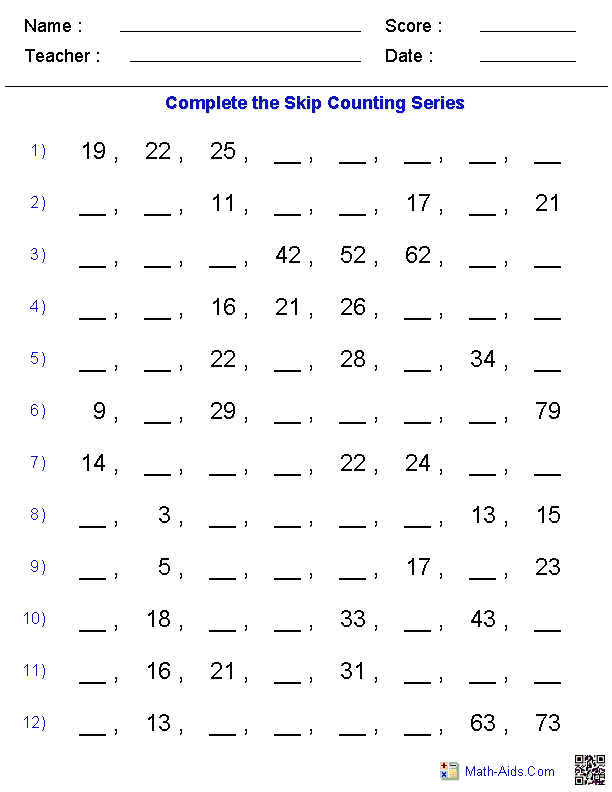##### Subtraction WorksheetsMath Worksheets##### Time WorksheetsMath Worksheets##### Venn Diagram WorksheetsMath Worksheets##### Word Games WorksheetsMath Worksheets##### Word Problems WorksheetsMath Worksheets